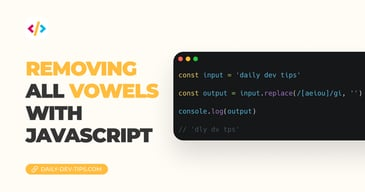Subscribe

# JavaScript basics logical operators

••••••• + 1 devs liked it

✍️

Exploring the three JavaScript logical operators

26 Aug, 2021 · 2 min read

In today's article, we'll be looking at JavaScript logical operators. JavaScript comes with three logical operators being `and`, `or` and `not`.

Check out the below table of the basic use cases.

OperatorLogicExample
`&&`And`a = true && b = false`
`||`Or`a = true || b = false`
`!`Not`let a = true`
`!a // false`

Let's have a more detailed view of each of these logical operators in JavaScript.

The `and` operator can be used to assess if two expressions are met.

The syntax is as follows:

``expression && expression;``

Some examples might be:

``````const a = true;
const b = 5;
a === true && b > 3;
// true``````

The return will always be an evaluation in the form of a boolean. We are returning either true if both expressions are met or false when one or both fails.

The operator is often used with a if...else statement to perform an action based on the logic.

Much like the `and` operator, we can also use the `or` operator, which is used by placing two pipes like this: `||`. This operator is used to evaluate if both or one of the expressions is met.

``expression || expression;``

Let's say we want to check if a is true or b is greater than 3. We don't need both to be truthy, just one.

``````const a = true;
const b = 1;
a === true || b > 3;
// true``````

The above example will still return true since it will succeed to be correct.

This is a bit of a funny one, as it is used to invert the value of a boolean.

So let's say we have a true boolean and want to convert it to false:

``````let a = true;
!a;
// false``````

However, using this in an if statement will evaluate if the condition is NOT met.

``````let a = true;

if (!a) {
// It will never get here now
}``````

However, we mainly use this to convert a value to the opposite boolean value.

Tweet this tip
••••••• + 1 devs liked it

### Removing all vowels with JavaScript

3 Dec, 2022 · 2 min read### 10 games to learn JavaScript

2 Dec, 2022 · 3 min readJoin 2088 devs and subscribe to my newsletter

• 1000 articles written
• 2088 devs subscribed
• 529116 words written